### IMO Shortlist 2017 problem N1

Kvaliteta:
Avg: 0,0
Težina:
Avg: 6,0

For each integer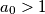$a_0 > 1$, define the sequence$a_0, a_1, a_2, \ldots$ for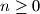$n \geq 0$ as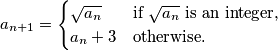Determine all values of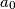$a_0$ such that there exists a number$A$ such that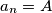$a_n = A$ for infinitely many values of$n$.

Izvor: https://www.imo-official.org/problems/IMO2017SL.pdf